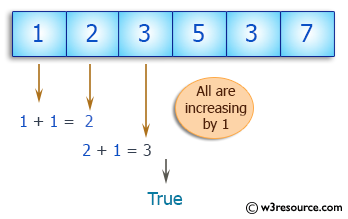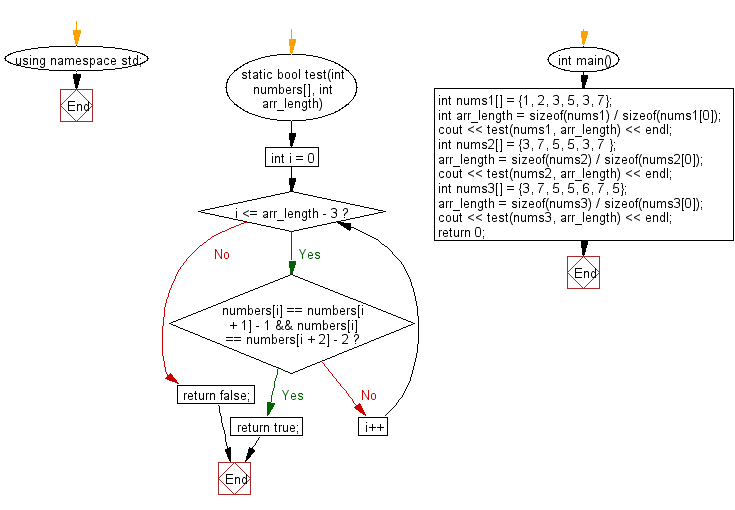﻿ C++ : Check an array contains 3 increasing adjacent elements# C++ Exercises: Check a given array of integers and return true if the array contains three increasing adjacent numbers

## C++ Basic Algorithm: Exercise-115 with Solution

Write a C++ program to check a given array of integers and return true if the array contains three increasing adjacent numbers.

Sample Solution:

C++ Code :

``````#include <iostream>
using namespace std;

static bool test(int numbers[], int arr_length)
{
for (int i = 0; i <= arr_length - 3; i++)
{
if (numbers[i] == numbers[i + 1] - 1
&& numbers[i] == numbers[i + 2] - 2)
{
return true;
}
}
return false;
}

int main()
{
int nums1[] = {1, 2, 3, 5, 3, 7};
int arr_length = sizeof(nums1) / sizeof(nums1);
cout << test(nums1, arr_length) << endl;
int nums2[] = {3, 7, 5, 5, 3, 7 };
arr_length = sizeof(nums2) / sizeof(nums2);
cout << test(nums2, arr_length) << endl;
int nums3[] = {3, 7, 5, 5, 6, 7, 5};
arr_length = sizeof(nums3) / sizeof(nums3);
cout << test(nums3, arr_length) << endl;
return 0;
}
``````

Sample Output:

```1
0
1
```

Pictorial Presentation:Flowchart:C++ Code Editor:

Contribute your code and comments through Disqus.

What is the difficulty level of this exercise?

﻿